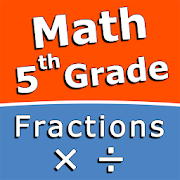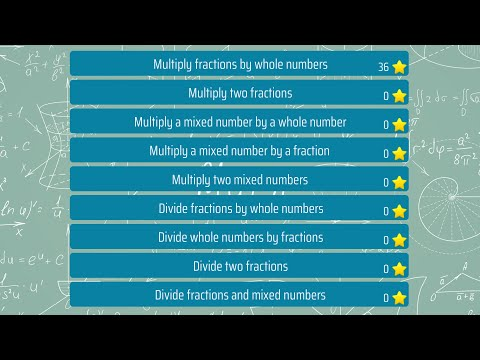# Multiply and divide fractions - 5th grade mathEveryoneIntuitive interface powered by handwriting input and a choice of three fun and engaging mini games in addition to a regular math trainer mode make our app stand out from the crowd of generic math learning apps.

With Multiply and divide fractions - 5th grade math skills you can practice and improve the following math skills:
- Multiply fractions by whole numbers
- Multiply two fractions
- Multiply a mixed number by a whole number
- Multiply a mixed number by a fraction
- Multiply two mixed numbers
- Divide fractions by whole numbers
- Divide whole numbers by fractions
- Divide two fractions
- Divide fractions and mixed numbers
Collapse

## Reviews

Review Policy

Eligible for Family LibraryEligible if bought after 7/2/2016. Learn More
Updated
May 24, 2020
Size
Varies with device
Installs
1,000+
Current Version
Varies with device
Requires Android
Varies with device
Content Rating
Everyone
Permissions
Offered By
Sergey Malugin
Developer
Taubenbrunnen 6 37120 Bovenden Germany